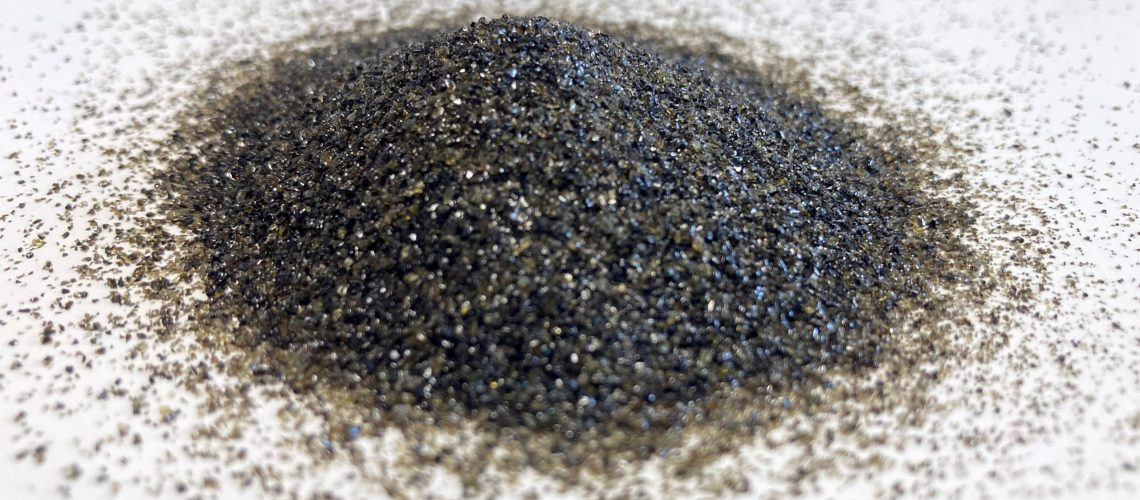# How much abrasives do you need for a grit blasting project?

This depends on the type of abrasive, the amount of cycles that the abrasive can be re-used, and the density of the abrasive. With a tank volume of 200 litres, blasting can be done for about 45 minutes, but as the density of the abrasives varies, so does the number of kilograms of abrasive used in that time. The net consumption of corundum and garnet sand can be calculated as follows:

The units for this formula are:

Tankvolume = m3
Time = hours
density = kg/dm3
Recycling rate= number of passages that the same grit particle goes through the nozzle of the system

For Grittal in practice, the net consumption is at least 30 times smaller than the net consumption of Corundum. The following table summarizes the density, recycling steps and the net usage of abrasives